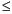Functions and CALL Routines

# LCOMB Function

Computes the logarithm of the COMB function, which is the logarithm of the number of combinations of n objects taken r at a time.
 Category: Combinatorial

## Syntax

 LCOMB(n,r)

### Arguments

n

is a non-negative integer that represents the total number of elements from which the sample is chosen.

r

is a non-negative integer that represents the number of chosen elements.

 Restriction: rn

The LCOMB function computes the logarithm of the COMB function.

The following statements produce these results:

SAS Statements Results
x=lcomb(5000,500);

put x;

1621.4411361

y=lcomb(100,10);

put y;

30.482323362

 Functions:Previous Page | Next Page | Top of Page